Courses

Introduction to Electric Charges Notes | Study Physics For JEE - Class 12

Class 12: Introduction to Electric Charges Notes | Study Physics For JEE - Class 12

The document Introduction to Electric Charges Notes | Study Physics For JEE - Class 12 is a part of the Class 12 Course Physics For JEE.
All you need of Class 12 at this link: Class 12

What is Electric Charge?

Electric charge is the basic physical property of matter that causes it to experience a force when kept in an electric or magnetic field.

• An electric charge is associated with an electric field and the moving electric charge generates a magnetic field. A combination of electric and magnetic fields is known as the electromagnetic field.
• Interaction of the charges generates an electromagnetic force which is the foundation of Physics. Electric Charge comes from the name of electricity, which is coined from the Greek word 'elektron' meaning amber.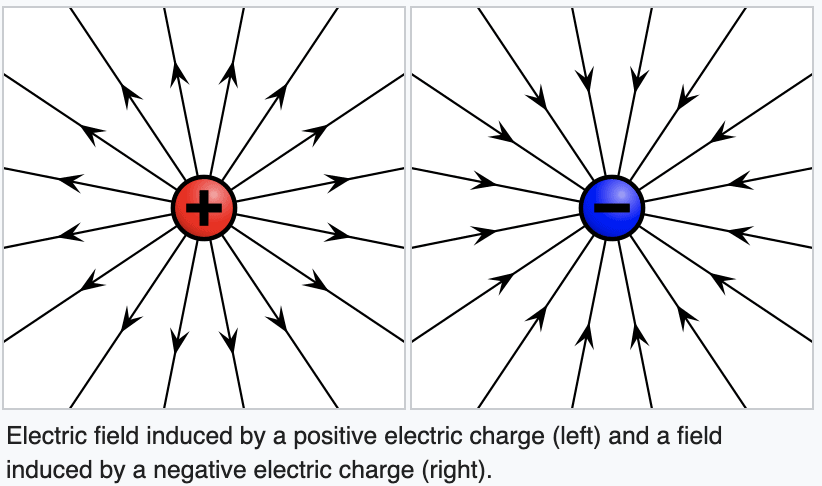Types of Electric Charges

Two kinds of electric charges are there:

1. Positive(+) charge
2. Negative(-) charge

Positive Charge: When an object has a positive charge it means that it has more protons than electrons.

Negative Charge: When an object has a negative charge it means that it has more electrons than protons.

When there is an identical number of positive and negative charges, the negative and positive charges would cancel out each other and the object would become neutral.

• Atoms are the building blocks of the universe. Whatever you see around you can be divided into smaller and smaller parts until you finally reach a part you cannot divide further. This building block is what we call an Atom.
Inside an atom are protons, electrons and neutrons. Out of the three, electrons and protons fit the definition of an electric charge.
• The protons are positively charged, the electrons are negatively charged, and the neutrons are neutral. A majority of the mass of the atom is concentrated into a very tiny space in the centre called the nucleus and the electrons revolve around this heavy nucleus.
• This means that electrons are held very loosely compared to protons. Therefore from henceforth the movement of charges here will be restricted to the movement of electrons. Since the atoms are made up of protons and electrons, we can safely conclude that all things are made up of electric charges.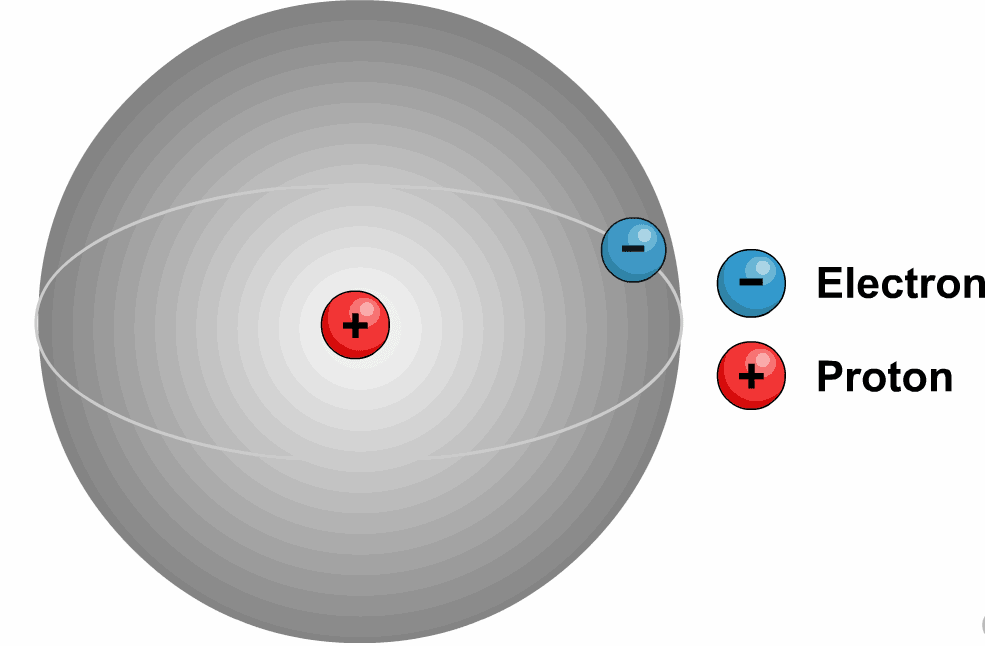Note:

Quantity of negative charge on an electron = quantity of positive charge on a proton.

• The charge of one proton is equal in strength to the charge of one electron. When the number of protons in an atom equals the number of electrons, the atom itself has no overall charge, it is neutral.
• Charge of a material body or particle is the property (acquired or natural) due to which it produces and experiences electrical and magnetic effects. Some of the naturally charged particles are electrons, proton, α-particle etc.
• Benjamin Franklin introduced the concept of positive and negative charges.

Try yourself:If a body has positive charge on it, then it means it has

Is Electric Charge a Vector Quantity?

• No, electric charge is a scalar quantity
• Apart from having a 'magnitude' and 'direction', for a quantity to be termed a vector (which we will study in detail later) it should also obey the laws of vector addition such as triangle law of vector addition and parallelogram law of vector addition, only then the quantity is said to be a vector quantity.
• In the case of an electric current, when two currents meet at a junction, the resultant current of these will be an algebraic sum and not the vector sum. Therefore, an electric current is a scalar quantity although it possesses magnitude and direction.

How to measure an Electric charge?

The electric charge is measured using a coulomb.

“One coulomb is the quantity of charge transferred in one second.”

Mathematically, the definition of a coulomb is represented as:

Q = I.t

In the equation, Q is the electric charge, I is the electric current and t is the time.

Unit of Electric Charge

A charge is a derived physical quantity. The charge is measured in coulomb in the S.I. unit.

In practice we use:

• millicoulomb mC (10-3 C)
• microcoulomb μC (10-6 C)
• nanocoulombs nC (10-9 C)
• C.G.S unit of charge = electrostatic unit = esu
• 1 coulomb = 3 × 109 esu of charge
• Dimensional formula of charge = [M0L0T1A1]

Note:

• Charge of a single electron = -1.602 × 10-19 C
• Charge of a single proton = + 1.602 × 10-19 C
• Charge of a single neutron = 0 C

Properties of Electric Charge

1. Like charges repel each other and unlike charges attract each other. (As Electric Charge comes in two varieties, which are called “plus” and “minus”.)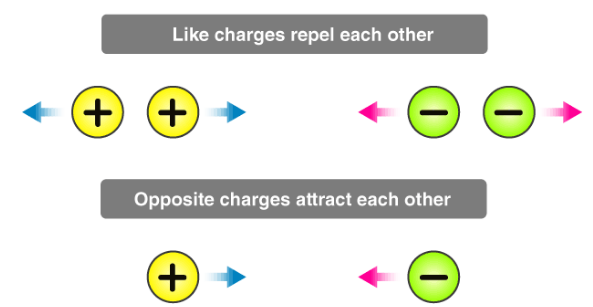2. Electric Charge is a scalar quantity: It follows scalar laws of operations, i.e. it adds algebraically and represents the excess of electrons in a negatively charged atom or a deficiency of electrons in a positively charged atom.

3. A charge is transferable: Electric charge can be transferred from one body to another, but there is a restriction to the charge transfer. Only electrons are transferred from one body to another because protons are tightly bound to the nucleus of every atom. Hence, the body which loses electrons in the transfer becomes positively charged, and the body which receives electrons becomes negatively charged.

• A neutral body has a number of electrons = number of protons
• A positively charged body has a number of electrons < number of protons
• A negatively charged body has a number of electrons > number of protons.

4. Charge is always conserved: In an isolated system, the total charge (sum of positive and negative) remains constant whatever charge transfer takes place in the system internally. It is called the principle of charge conservation.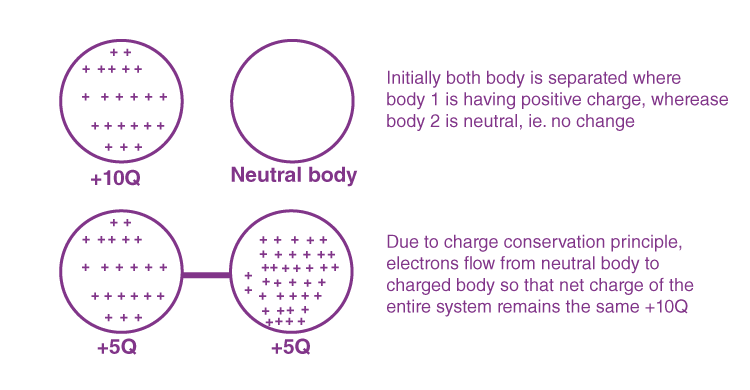Conservation of charge

5. Charge is quantized: Charge on anybody always exists in integral multiples of a fundamental unit of electric charge. This unit is equal to the magnitude of the charge on one electron (1e = 1.6 × 10-19 C). So charge on anybody Q = ± ne, where n is an integer and e is the charge on a single electron. This was proved by Millikan's oil drop experiment.

• Recently, the existence of particles of charge +(2/3) e and -(1/3) e has been postulated. These particles are called quarks, but still, this is not considered as the quantum of charge because these are unstable (They have a very short span of life.)

6. Charge is always associated with mass: Yes! Electrons, Protons and Neutrons also have masses.

Their value is determined, experimentally, to be following:
Mass of an electron = 9.109 × 10-31 Kg = 5.49 × 10-4 amu
Mass of a proton = 1.6726 × 10-27 Kg = 1.007 amu
Mass of a neutron = 1.6749 × 10-27 Kg = 1.008 amu

• It is recommended to remember these values in Kg (SI units). Also, please note that the mass of a neutron is slightly greater than the mass of a proton.
• This also shows that the mass of a negatively charged body is greater than the mass of a positively charged identical body as it would have an excess number of electrons than the positively charged bodies.

7. Charge is relativistically invariant: This means that charge is independent of the frame of reference, i.e., the charge on a body does not change whatever be its speed. This property is worth mentioning as in contrast to charge, the mass of a body depends on its speed and increases with an increase in speed. You will be exposed to this property later when you will learn The Special Theory of Relativity.

8. A charge at rest produces an only an electric field around itself: A charge at rest creates a region of influence called an Electric Field around itself in space.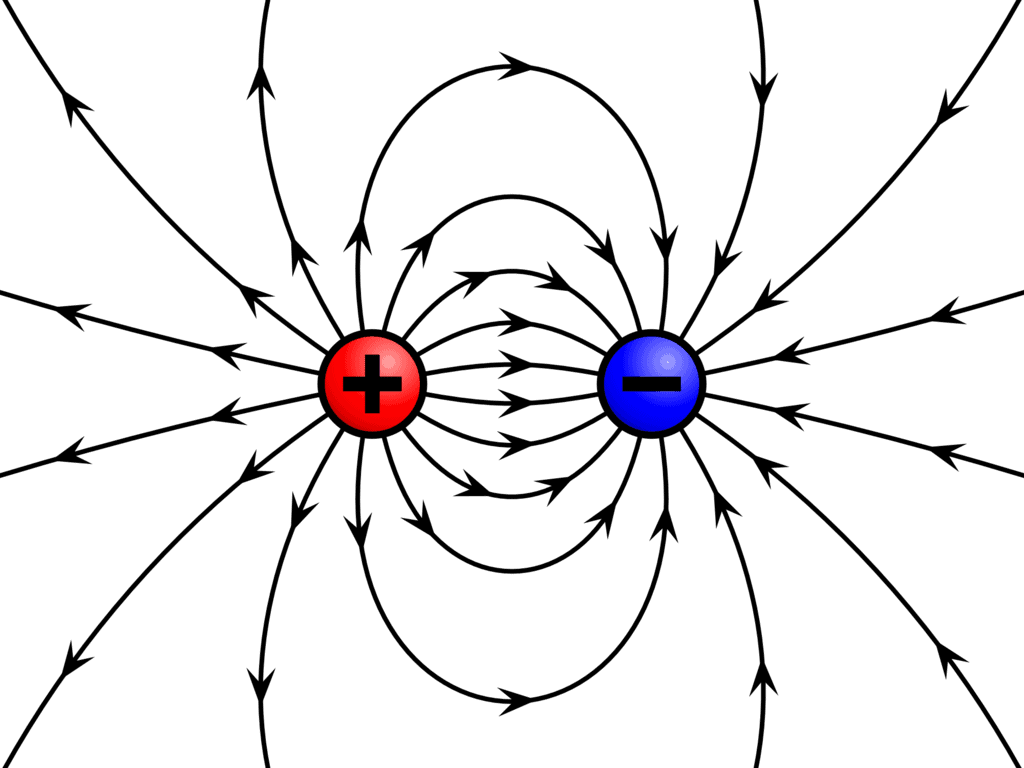Electric field lines

• While a charge having uniform motion (constant velocity) produces electric as well as the magnetic field around itself.
• Accelerated charges produce a special combination of electric and magnetic fields called electromagnetic waves. We will study the electric field in detail in the coming section.

Try yourself:Five balls marked a to e are suspended using separate threads. Pairs (b, c) and (d, e) show electrostatic repulsion while pairs (a, b), (c, e) and (a, e) show electrostatic attraction. The ball marked a must be

Activity to Understand Charges

• Have you felt the sudden painful jolt you get when you brush someone’s arm? If you have a woollen blanket then you should definitely do this, switch off the light and brush your clothes with the woollen blanket.
• The number of sparks that go off will amaze you. Believe it or not, this very phenomenon is also responsible for all the lightning strikes on our planet. So what is it? It is known as Static Electricity.
• Static electricity can be a nuisance or even a danger. The energy that makes your hair to stand on end can also damage electronic equipment’s and cause explosions.The comb attracting small pieces of paper with static electricity

What is Static Electricity?

Static electricity refers to an imbalance between the electric charges in a body, specifically the imbalance between the negative and the positive charges on a body.

• The imbalance in the charge is introduced by physical means. One of the most common causes of static electricity is contact between solid objects. It was mentioned earlier that the movement of protons is not possible and the only movement of electric charge seen in static electricity is electrons.
• Electrons in materials are held extremely loosely meaning that they can be exchanged through simple contact like rubbing.
• The image below is an example of rubbing a glass rod with silk which causes static electricity. When two objects are rubbed together to create static electricity, one object gives up electrons and becomes more positively charged while the other material collects electrons and becomes more negatively charged.
• We should keep in mind that the rules such as like charges repel and unlike charges attract is applicable here.

1. How are electric charges distributed within the atom?
Subatomic particles carry electric charges. Electrons carry the negative charge and protons carry the positive charge in the nuclei of atoms.

2. Why is an electric charge a scalar quantity?
When two currents meet at a junction, the resultant current of these will be an algebraic sum and not the vector sum. Therefore, an electric current is a scalar quantity.

3. When will an electric charge be negative?
When the matter has more electrons than protons then it is said to have a negative charge.

4. When will an electric charge be positive?
When the matter has more number of protons than electrons, then it is said to have a positive charge.

5. What is the unit for measuring electric charge?
Coulomb is the unit for measuring electric charge

Concept-Based Questions

Q.1. Why have we defined only two types of charges? Why not three or more?

Only two kinds of electric charges exist because any unknown charge that is found experimentally to be attracted to a positive charge is also repelled by a negative charge. No one has ever observed a charged object that is repelled by both a positive and a negative charge.

Q.2. What happens

(a) When two like charges are brought together?

(b) When two, unlike charges, are brought together?

(a) When two like charges are brought together, they repel each other with an electrostatic force.

(b) When two, unlike charges, are brought together, they attract each other with an electrostatic force.

Q.3. What does neutral in electric charge mean?

Neutral does not refer to any third type of charge. It is the absence of any excess or deficiency of electrons in a body, i.e. the number of electrons = number of protons.

Q.4. Does the mass of the body get affected while charging?

Yes, the mass of the body gets affected because electrons have a definite mass, so the mass of the body slightly increases when it gains electrons while the mass decreases when it loses electrons.

Q.5. Two identical metallic spheres of exactly equal masses are taken. One is given positive charge q coulombs and the other an equal negative charge. Are their masses after charging equal?

No, A body is positively charged due to the deficit of electrons while the negative charge is due to a surplus of electrons. Hence, the mass of the negatively charged sphere will be slightly more than that of the positively charged sphere.

Q.6. During a nuclear reaction, what happens to electric charge?

In the event of a nuclear reaction, the electric charge is conserved considering an isolated system. This is true for any nuclear or chemical reaction. In a nuclear reaction, the parent nuclei undergo a transformation into daughter nuclei, but the total algebraic charge remains constant.

Q.7. Explain the statement: ‘For a body, an electric charge is quantized.

Considering a particular body, ‘electric charge is quantized’ refers to the number of electrons which can be transferred from that body to another. It should be noted that charges don’t get transported in fractions. Therefore, the overall charge on a body is simply an integral multiple of charge on an electron.

The document Introduction to Electric Charges Notes | Study Physics For JEE - Class 12 is a part of the Class 12 Course Physics For JEE.
All you need of Class 12 at this link: Class 12Use Code STAYHOME200 and get INR 200 additional OFF Use Coupon Code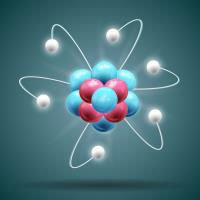Physics For JEE

187 videos|516 docs|256 tests

Track your progress, build streaks, highlight & save important lessons and more!

,

,

,

,

,

,

,

,

,

,

,

,

,

,

,

,

,

,

,

,

,

;